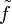# Splitting implies characters form a basis for space of class functions

This fact is related to: linear representation theory
View other facts related to linear representation theoryView terms related to linear representation theory |

## Statement

Suppose$G$ is a finite group, and$k$ is a splitting field for$G$; in other words, every representation of$G$ over$k$ is completely reducible and every representation irreducible over$k$ is irreducible over any field extension of$k$.

Then the characters of the irreducible representations of$G$ over$k$, span the$k$-vector space of class functions on$G$ with values in$k$. In fact, they form an orthonormal basis under the inner product:$\langle f_1, f_2 \rangle := \frac{1}{|G|} \sum_{g \in G} f_1(g) f_2(g^{-1})$

In the case where$k = \mathbb{C}$, we can also use the Hermitian inner product:$\langle f_1, f_2 \rangle := \frac{1}{|G|} \sum_{g \in G} f_1(g) \overline{f_2(g)}$

(although the two inner products are not the same (one is symmetric bilinear, the other is Hermitian) their values on pairs of characters are the same, because trace of inverse is complex conjugate of trace).

## Facts used

1. Character orthogonality theorem (we use this both directly and in the form of a slight variant/modification of the orthogonal projection formula, though we don't call it out explicitly in the text of the proof).
2. Schur's lemma
3. Maschke's averaging lemma

## Proof

We present the proof for splitting fields in general using the symmetric bilinear form. A similar proof works if we use the Hermitian inner product instead.

### Any class function orthogonal to all irreducible characters must be identically zero

Given: A finite group$G$, a splitting field$k$ for$G$. A class function$f:G \to k$ such that$\langle f, \chi \rangle_G = 0$ for every irreducible character$\chi$.

To prove:$f$ is the zero function.

Proof:

Step no. Assertion/construction Facts used Given data used Previous steps used Explanation
1 For any irreducible representation$\varphi$ of$G$ over$k$, the matrix$\sum_{g \in G} f(g)\varphi(g^{-1})$ defines a homomorphism of representations from$\varphi$ to itself, viewed as a representation over the algebraic closure$\overline{k}$.$f$ is a class function. PLACEHOLDER FOR INFORMATION TO BE FILLED IN: [SHOW MORE]
2 For any irreducible representation$\varphi$ of$G$ over$k$, the matrix$\sum_{g \in G} f(g)\varphi(g^{-1})$ is a scalar matrix. Fact (2)$k$ is a splitting field. Step (1) [SHOW MORE]
3 The matrix$\sum_{g \in G} f(g)\varphi(g^{-1})$ is the zero matrix for any irreducible representation of$G$ over$k$.$f$ is orthogonal to the character of every irreducible representation. Step (2) [SHOW MORE]
4 The matrix$\sum_{g \in G} f(g)\rho(g^{-1})$ is the zero matrix for any representation of$G$ over$k$.$k$ is splitting -- every representation of$G$ over$k$ splits completely as a sum of irreducible representations. Note that by Fact (3), the complete reducibility follows from the characteristic not dividing the order of$G$. Step (3) [SHOW MORE]
5 Setting$\rho$ as the regular representation (i.e., the permutation representation arising from the regular group action) we get$f(g) = 0$ for all$g$. Step (4) [SHOW MORE]

### Characters form a basis for the space of class functions

Step no. Assertion/construction Facts used Given data used Previous steps used Explanation
1 The characters of inequivalent irreducible representations are linearly independent on account of being an orthonormal set. In other words, if$a_1\chi_1 + a_2\chi_2 + \dots + a_r\chi_r = 0$ for distinct irreducible characters$\chi_i$ and$a_i \in k$, then all the$a_i$ equal$0$. Fact (1) [SHOW MORE]
2 In particular, this means that there can be at most finitely many irreducible representations up to equivalence (because they form a linearly independent subset in a finite-dimensional vector space). Step (1)
3 For any class function$f$, consider$\tilde{f} = f - \sum_{i=1}^r \langle f, \chi_i \rangle \chi_i$ where$\chi_1,\chi_2 ,\dots,\chi_r$ are all the irreducible characters of$G$. Then$\tilde{f}$ is a class function orthogonal to all irreducible characters. Fact (1) [SHOW MORE]
4$\tilde{f} = 0$, so$f = \sum_{i=1}^r \langle f, \chi_i \rangle \chi_i$ for any class function$g$. Step (3), Previous half of proof which shows that there any class function orthogonal to all irreducible characters is zero Step-combination direct.
5 The characters form a basis (in fact, an orthonormal basis) for the space of class functions. Steps (1), (4) Step (1) shows they are linearly independent, Step (4) shows that they span the space. Together, this means they are a basis.